# OECD Discriminatory family code data analysis 5 - Linear regression using R. Attitudes Towards Working Mother, Early Marriage and Per Capita GDPPhoto by Bjorn Pierre on Unsplash

This post is following of above post.
In this post, I will do linear regression analysis with R.

First, I make a data frame which have "atwm": Attitudes Towards Working Mothers only.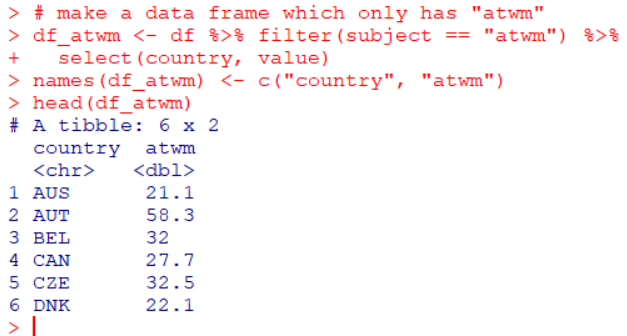Second, I make a data frame which have "em": Early Marriage only.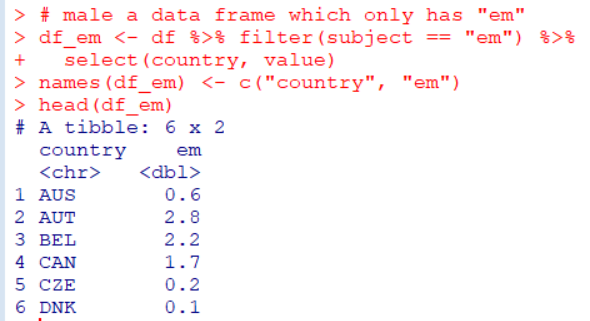Then, I merge those two data frames with merge() function.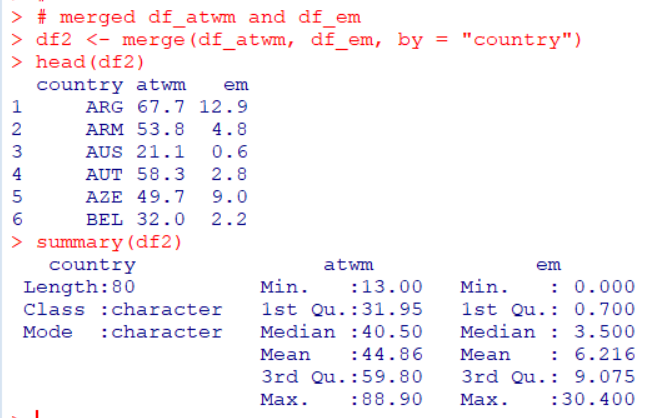Now we have 80 observations in df2.

Let's make a scatter plot for "atwm" and "em".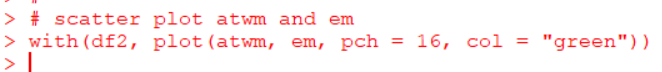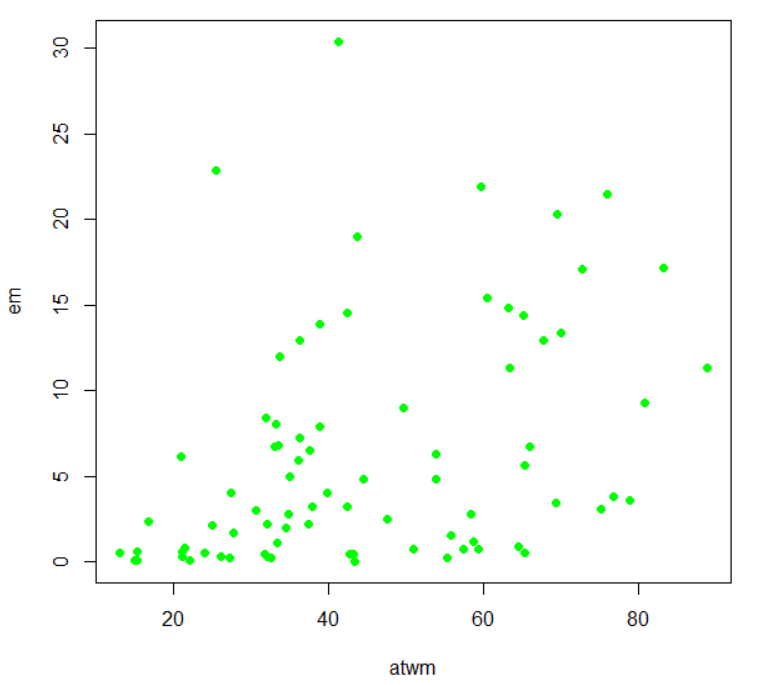I use lm() function for linear regression.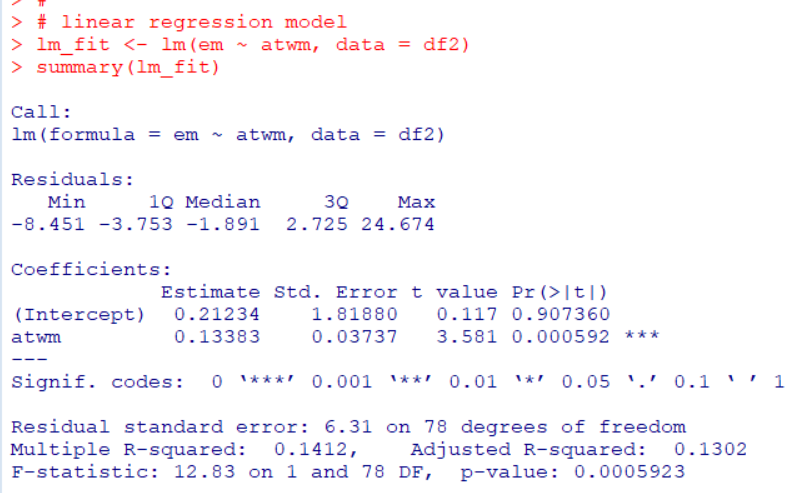p-value is less than 0.05, so I say this model is statistically significant.

Let's make plot for scatter plot and regression line.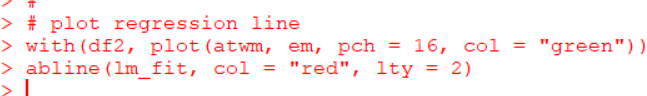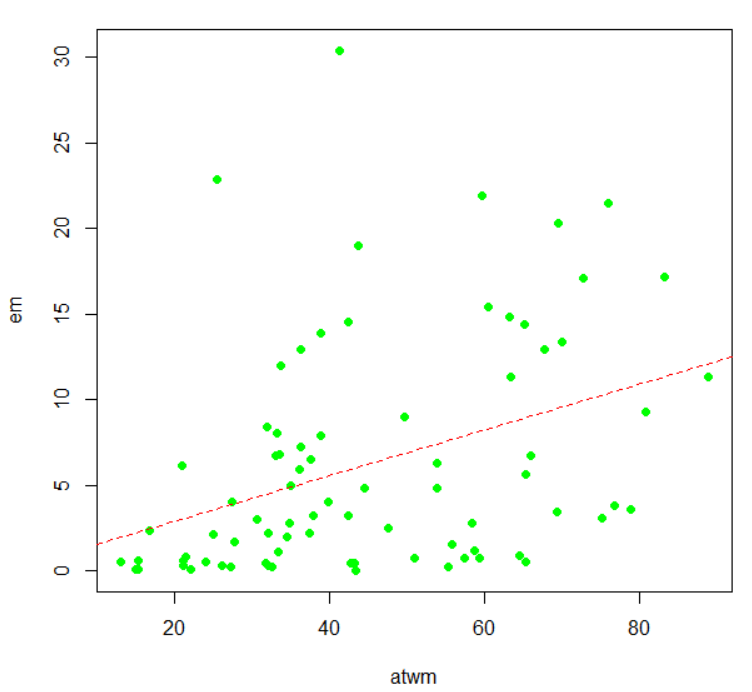We see the higer "atwm", the higher "em".

Let's test for Heteroskedasticity.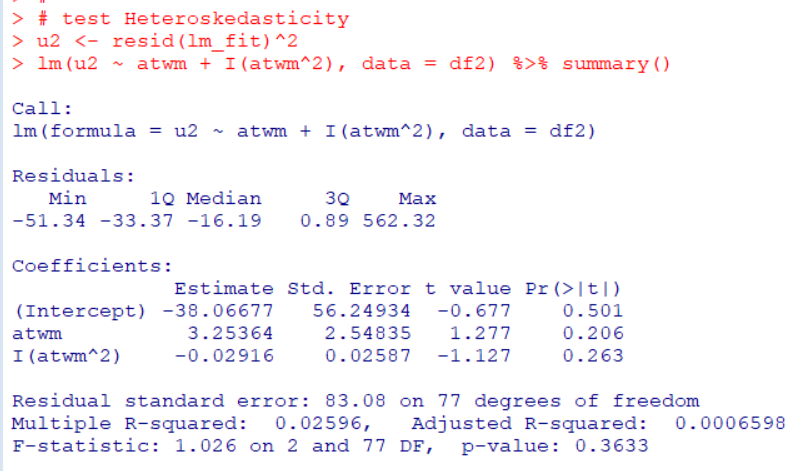atwm, I(atwm^2) and entire model's p-value are all greater than 0.05, 0.206, 0.263 and 0.363 respectively. So this model is not Heteroskedasticiy.

I have per capita GDP file which is downloaded from OECD web site.
I will load this GDP data file.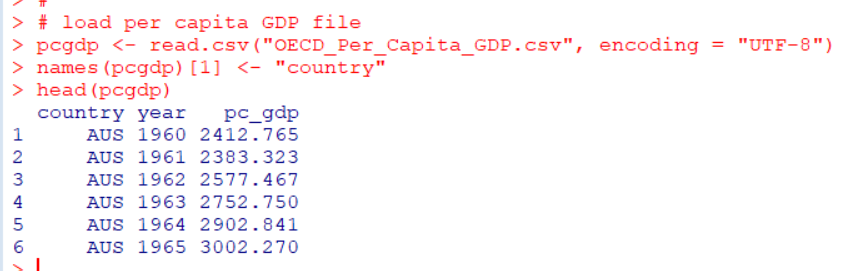I merge this pcgdp data frame and df2 data frame.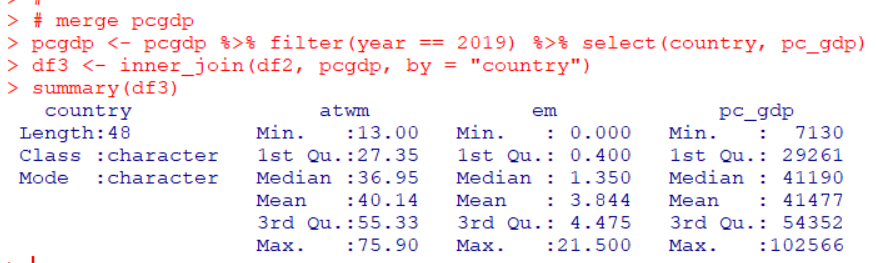I make log(pc_gdp) for linear regression analysis and make two scatter plots.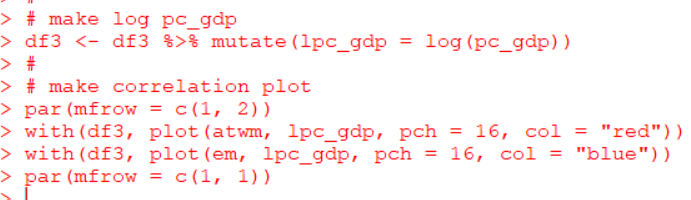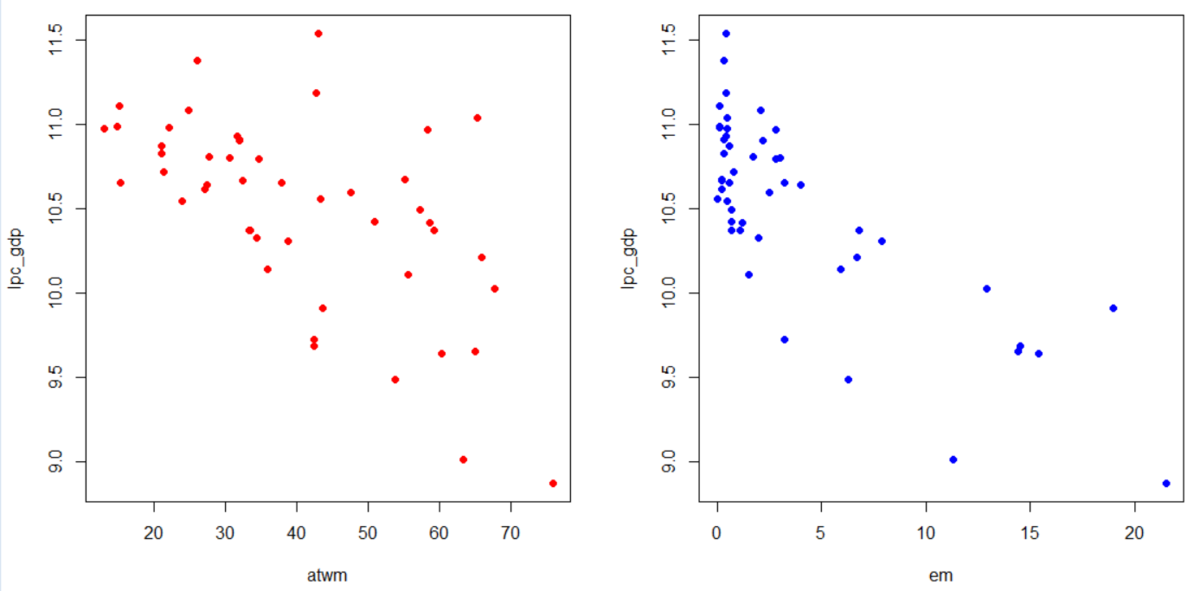I wee "atwm" and "em" have negative relationship to "lpc_gdp": log(per capita GDP).

So, the lower "atwm" and "em", the higher per capita GDP.

Let's do linear regression with lm() function.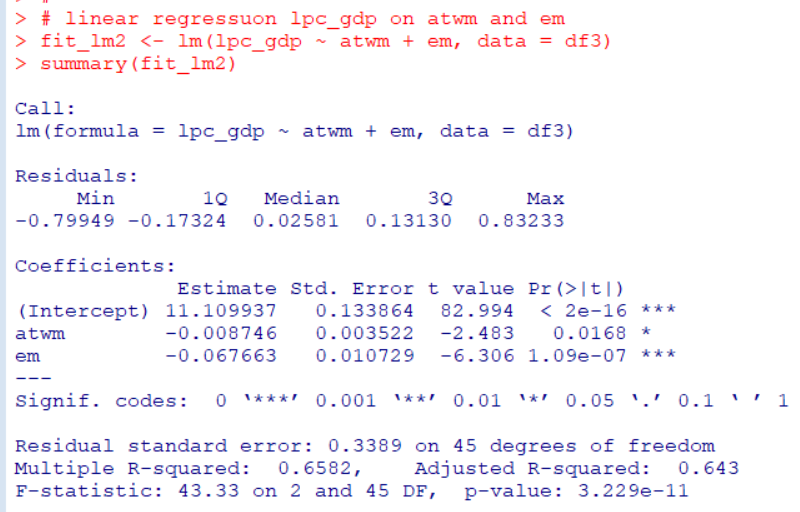"atwm" and "em" has statistically significant coefficient. The both coefficients signs are negative.

Lastly, let's make a 3D scatter plot with scatterplot3d() function in scatterplor3d package.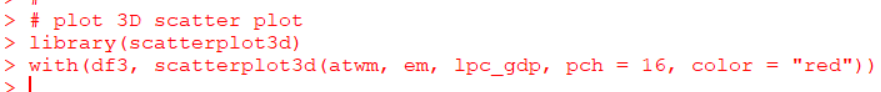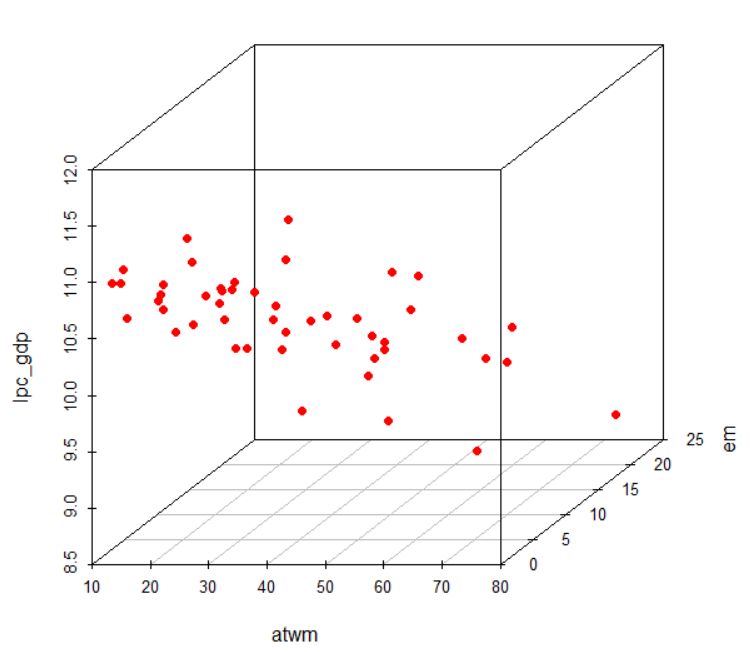That's it. Thank you!

Next post is